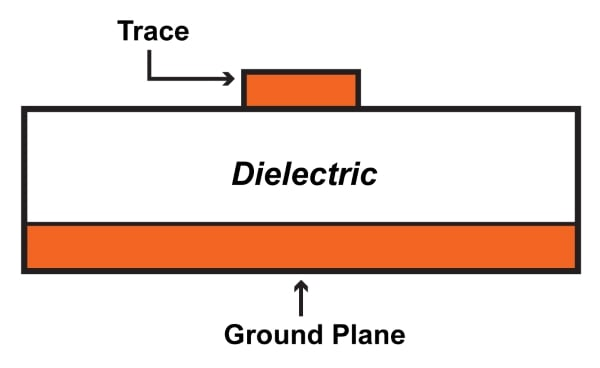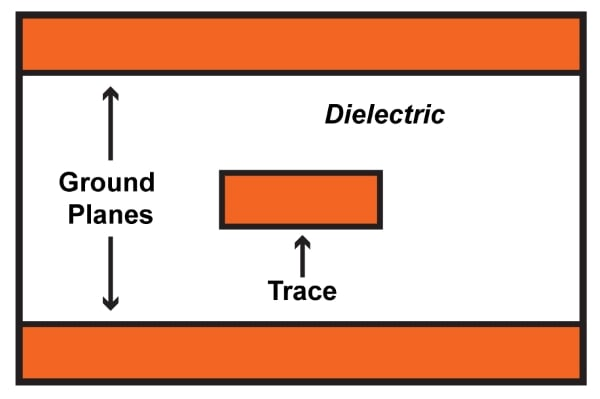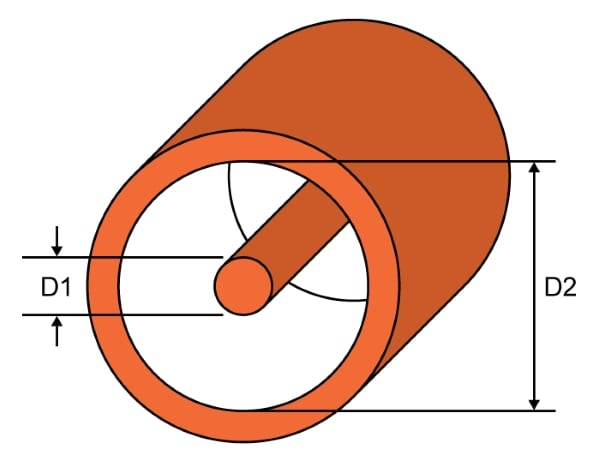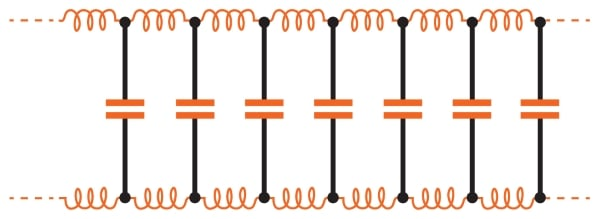Vol. Practical Guide to Radio-Frequency Analysis and Design
Chapter 3 Real-Life RF Signals

# What Is a Transmission Line?

## High-frequency interconnects require special consideration because they often behave not as ordinary wires but rather as transmission lines.

High-frequency interconnects require special consideration because they often behave not as ordinary wires but rather as transmission lines.

In low-frequency systems, components are connected by wires or PCB traces. The resistance of these conductive elements is low enough to be negligible in most situations.

This aspect of circuit design and analysis changes dramatically as frequency increases. RF signals do not travel along wires or PCB traces in the straightforward fashion that we expect based on our experience with low-frequency circuits.

### The Transmission Line

The behavior of RF interconnects is very different from that of ordinary wires carrying low-frequency signals—so different, in fact, that additional terminology is used: a transmission line is a cable (or simply a pair of conductors) that must be analyzed according to the characteristics of high-frequency signal propagation.

First, let’s clarify two things:

#### Cable vs. Trace

“Cable” is a convenient but imprecise word in this context. The coaxial cable is certainly a classic example of a transmission line, but PCB traces also function as transmission lines. The “microstrip” transmission line consists of a trace and a nearby ground plane, as follows:The “stripline” transmission line consists of a PCB trace and two ground planes:PCB transmission lines are particularly important because their characteristics are controlled directly by the designer. When we buy a cable, its physical properties are fixed; we simply gather the necessary information from the datasheet. When laying out an RF PCB, we can easily customize the dimensions—and thus the electrical characteristics—of the transmission line according to the needs of the application.

#### The Transmission Line Criterion

Not every high-frequency interconnect is a transmission line; this term refers primarily to the electrical interaction between signal and cable, not to the frequency of the signal or the physical characteristics of the cable. So when do we need to incorporate transmission-line effects into our analysis?

The general idea is that transmission-line effects become significant when the length of the line is comparable to or greater than the wavelength of the signal. A more specific guideline is one-fourth of the wavelength:

• If the interconnect length is less than one-fourth of the signal wavelength, transmission-line analysis is not necessary. The interconnect itself does not significantly affect the electrical behavior of the circuit.
• If the interconnect length is greater than one-fourth of the signal wavelength, transmission-line effects become significant, and the influence of the interconnect itself must be taken into account.

Recall that wavelength is equal to propagation velocity divided by frequency:

$$\lambda=\frac{v}{f}$$

If we assume a propagation velocity of 0.7 times the speed of light, we have the following wavelengths:

 1 kHz 210 km 1 MHz 210 m 1 GHz 210 mm 10 GHz 21 mm

The corresponding transmission-line thresholds are the following:

 1 kHz 52.5 km 1 MHz 52.5 m 1 GHz 52.5 mm 10 GHz 5.25 mm

So for very low frequencies, transmission-line effects are negligible. For medium frequencies, only very long cables require special consideration. However, at 1 GHz many PCB traces must be treated as transmission lines, and as frequencies climb into the tens of gigahertz, transmission lines become ubiquitous.

### Characteristic Impedance

The most important property of a transmission line is the characteristic impedance (denoted by Z0). Overall this is a fairly straightforward concept, but initially it can cause confusion.

First, a note on terminology: “Resistance” refers to opposition to any flow of current; it is not dependent on frequency. “Impedance” is used in the context of AC circuits and often refers to a frequency-dependent resistance. However, we sometimes use “impedance” where “resistance” would theoretically be more appropriate; for example, we might refer to the “output impedance” of purely resistive circuit.

Thus, it’s important to have a clear idea of what we mean by “characteristic impedance.” It is not the resistance of the signal conductor inside the cable—a common characteristic impedance is 50 Ω, and a DC resistance of 50 Ω for a short cable would be absurdly high. Here are some salient points that help to clarify the nature of characteristic impedance:

• Characteristic impedance is determined by the physical properties of the transmission line; in the case of a coaxial cable, it is a function of the inner diameter (D1 in the diagram below), the outer diameter (D2), and the relative permittivity of the insulation between the inner and outer conductors.• Characteristic impedance is not a function of cable length. It is present everywhere along the cable, because it results from the cable’s inherent capacitance and inductance.##### In this diagram, individual inductors and capacitors are used to represent the distributed capacitance and inductance that is continuously present throughout the length of the cable.

• In practice, a transmission line’s impedance is not relevant at DC, but a theoretical transmission line of infinite length would present its characteristic impedance even to a DC source such as a battery. This is the case because the infinitely long transmission line would perpetually draw current in an attempt to charge up its infinite supply of distributed capacitance, and the ratio of the battery voltage to the charging current would be equal to the characteristic impedance.
• The characteristic impedance of a transmission line is purely resistive; no phase shift is introduced, and all signal frequencies propagate at the same speed. Theoretically this is true only for lossless transmission lines—i.e., transmission lines that have zero resistance along the conductors and infinite resistance between the conductors. Obviously such lines do not exist, but lossless-line analysis is sufficiently accurate when applied to real-life low-loss transmission lines.

### Reflections and Matching

The impedance of a transmission line is not intended to restrict current flow in the way that an ordinary resistor would. Characteristic impedance is simply an unavoidable result of the interaction between a cable composed of two conductors in close proximity. The importance of characteristic impedance in the context of RF design lies in the fact that the designer must match impedances in order to prevent reflections and achieve maximum power transfer. This will be discussed in the next page.

### Summary

• An interconnect is considered a transmission line when its length is at least one-fourth of the signal wavelength.
• Coaxial cables are commonly used as transmission lines, though PCB traces also serve this purpose. Two standard PCB transmission lines are the microstrip and the stripline.
• PCB interconnects are typically short, and consequently they do not exhibit transmission-line behavior until signal frequencies approach 1 GHz.
• The ratio of voltage to current in a transmission line is referred to as the characteristic impedance. It is a function of the physical properties of the cable, though it is not affected by length, and for idealized (i.e., lossless) lines it is purely resistive.

#### Lessons in Electric Circuits

##### Volumes »
Published under the terms and conditions of the Design Science License